The electric force is very strong, but often not noticed. We will explain forces between charges, coulombs law, electric field strength and electric potential

1 The experiment - The dangling ball in an electric field

The plastic ball dangles on a string. In the electric field with a potential difference (p.d.) of 20 kV it accelerates very strong and you can observe amazing lightning. But why do we have this forces on a plastic ball?

The answer: The force is a result of different charges.

Coulomb wanted to know it more presicely. He observed that force can be attractive or repulsive. Since there are two different types of charge - positive and negative - he found out: different charges attract each other, identical charges repel.

2 Coulombs law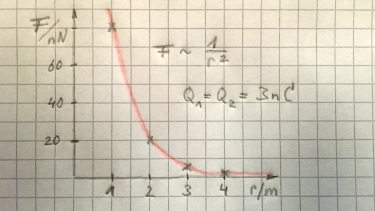Coulomb observed two charges by experiment and identified that the force is

• directly proportional to each charge $Q_1$ and $Q_2$,
• inversly proportional to the square of their distance $r$.

$$F=\frac{k\cdot Q_1 Q_2}{r^2}$$

The value for the constant of proportionality $k$ is $9.0 \cdot 10^9~Nm^2/(As)$. In vacuum or air it is written to $k=\frac{1}{4\pi \epsilon_0}$, where $\epsilon_0=8.85 \cdot 10^{-12}As/(Vm)$ is the permittivity of free space. Any other insulating material has a permittivity $\epsilon$ greater than $\epsilon_0$.

3 Electric fields

During thunderstorm we observe lightning. We say there is an elctric field around the lightning. It is possible to see the shape of an electric field with castor oil and semolina grain as shown and a p.d. of 20 kV. Field lines always enter the surface vertically, have a direction and as stronger the field the more dense the lines.

We introduce the electric field strength $E$ which just tells us the voltage drop per meter distance or the force excerted on a charge.

$$E=\frac{V}{d}=\frac{F}{Q}~~~~[E]=\frac{V}{m}=\frac{N}{As}$$
4 Electric Potential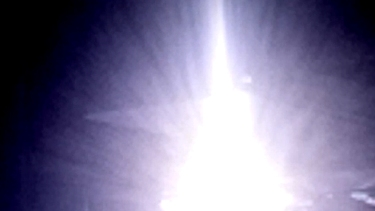During thunderstorm we have $E=200,000~V/m$. This means at an altitude of 1000 m an electric potential of $200,000,000~V$.

To ionize air we need $E = 3,000,000~V/m$. If the humidity increases this value decreases. Further more if the distance between clouds and earth decreases the electric field strength increases. So the air will ionize and for 10 ms a very high current of around $10,000~A$ will flow.

So we can estimate the energy of lightning:

$$E_{\text{el}}=V \cdot I \cdot t=5500~kWh$$A car in an electric field is a save place.

Try the following experiment: You enter a square of 2x2 m and assume you are an electron. The next person (also negative) enters the square. Just try to behave like two identical charges. The third person will enter the square. Where is the positioning of everybody? Not sure, yet? Try a fourth and fifth person.

If a lightning hits the car the electrons will flow through the metal cage of the car. Since electrons repel each other they stay at the outside of the car. As long as you are inside the car and you do not touch the outside, nothing will happen.

6 Electron beamsFast moving beams of electrons can be generated by an electron gun. Thermionic emission is generated by a heated cathode where electrons can escape. The electrons are accelerated by the positive anode. As the electrons are accelerated they loose potential energy, but win kinetic energy.

$$1/2mv^2=eV$$

Some elctrons pass through the anode and can be deflected by another electric field.

$$F=eE=e\frac{V}{d}$$

#### Question 1 force on charge

Which drawing is correct?

#### Question 2 Coulombs law

 $Q_1$ /nC $Q_2$ /nC $r$ /m $F$ /nN 3 3 1 3 3 2 3 3 3 3 6 3

What is the size of the force when

1. the charges are identical?
2. the charges are different?
3. one charge is doubled but the distance stays the same?
 $Q_1$ /nC $Q_2$ /nC $r$ /m $F$ /nN 3 3 1 80,9 3 3 2 20,2 3 3 3 8,99 3 6 3 17,98#### Experiment 1 shape of an electric field

idea by B. Höger, Tettnang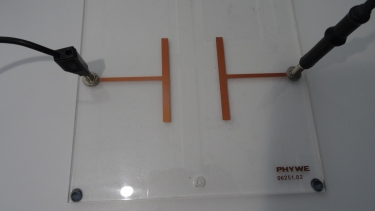All you need is castor oil and semolina grain as shown and a p.d. of 20 kV.

We cover the bottom of a glass with castor oil and spread a thin layer of some semolina grain. Now we put the glass on top of the capacitor and turn the high potential difference on.

hint: Just mix the semolina grain with some ink.

• How is the shape for a uniform electric field?

• How is the shape for a non uniform field?

• Which properties does the shape of the electric field have?

1. Shape of uniform field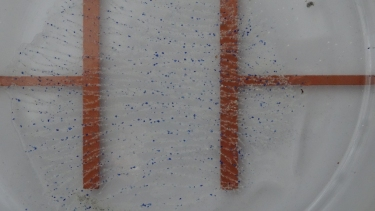2. Shape of non uniform field3. properties of the shape of the electric field:
The electric field lines enter vertically, they have a direction and the greater the electric field strength the more dense they are.

#### Question 3 ionisation

A spark can pass through air when the electric field strength is 3,000,000 V/m.

1. The gap between a human and the car is 2 mmm. What p.d. is needed to produce this spark.
2. An overhead electricity cable reaches a maximum voltage of 400,000 V. How close could you get to the cable before receiving an electric shock?
3. A fuse interrupts a power circuit with 230 V. How long is the distance, when the spark will be erased?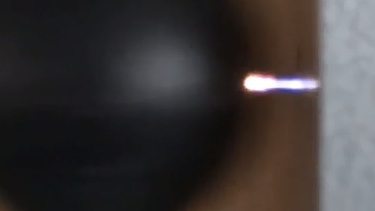1. potential difference p.d.: $V=E\cdot d=3,000,000~V/m \cdot 2\cdot 10^{-3}~m=6000~V$
2. distance: $d=\frac{V}{E}=\frac{400,000~V}{3,000,000~V/m}= 0.13~m =13~cm$
3. distance: $d=\frac{V}{E}=\frac{230~V}{3,000,000~V/m}= 76.7 \cdot 10^{-6}~m$

### Relax ...

Struck by lightning in a car.

#### Question 4 Charged oil drop

A negativley charged oil drop with a mass of $2.6\cdot 10^{-17}~$kg is held stationary in an electric field with a p.d. of 19 V in Millikan's experiment.

1. What are the two forces acting on the drop and in which direction do they act?
2. If the two forces balance the oil drop is held stationary. Calculate the charge on the oil drop.
1. Gravity (down) and Electrostatic force (up)
2. $$mg=QE=Q\frac{V}{d}$$ $$Q=1,6\cdot 10^{-19}~C$$